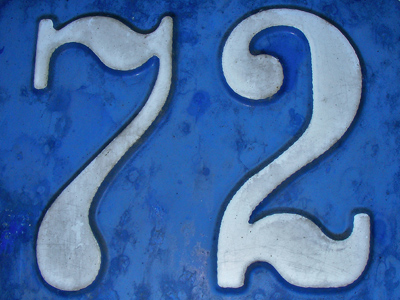72 is a multiple of 6, 8 and 12.

# Multiples (Very Easy)

The times tables that you have learnt will help you in this multiples quiz. A 'multiple' is a number that can be divided exactly by another given number, e.g. 6 is a multiple of 3 because 3 divides 6 exactly two times. In addition, 3.4 is a multiple of 1.7 because 1.7 divides 3.4 exactly two times.

In this 11-plus Maths quiz we shall only be dealing with whole numbers BUT it's a good idea to keep a lookout for multiples when you work with decimals. As a fun experiment see how many numbers you come across today, write them down and find out how many multiples you can find. Doing these kinds of exercises will really help with your knowledge of numbers and Maths.

It's a really easy quiz, so if you don't get 10 out of 10 first time play it again until you do. Enjoy yourself!

1.
Which of the four choices is a multiple of ALL the numbers given?
5, 10, 15, 25
105
125
50
150
105 isn't divisible by 10 or 25, 125 isn't divisible by 10 or 15 and 50 isn't divisible by 15
2.
Which of the four choices is a multiple of ALL the numbers given?
4, 16, 32
48
80
52
64
48 isn't divisible by 32 and neither is 80. 52 is only divisible by 4
3.
Which of the four choices is a multiple of ALL the numbers given?
1, 2, 3, 4, 6
12
18
16
14
A good strategy is as follows: find a likely multiple and check to see if one of the given numbers DOESN'T divide it. If you can't find one, then that's your multiple. If you do find one, then that's NOT your multiple, e.g. 4 doesn't divide 14
4.
Which of the four choices is a multiple of ALL the numbers given?
5, 15, 25
100
75
50
125
All the incorrect answers are not divisible by 15
5.
Which of the four choices is a multiple of ALL the numbers given?
2, 4, 6, 8
48
42
36
40
42 isn't correct because 4 and 8 don't divide into whole numbers. 36 isn't correct because 8 doesn't divide. 40 isn't correct because 6 doesn't divide
6.
Which of the four choices is a multiple of ALL the numbers given?
3, 4, 6, 8
24
28
26
32
28 is only divisible by 4, 26 isn't divisible by any of them and 32 isn't divisible by 3 or 6
7.
Which of the four choices is a multiple of ALL the numbers given?
3, 5, 7
35
15
105
21
35 isn't divisible by 3, 15 isn't divisible by 7 and 21 isn't divisible by 5
8.
Which of the four choices is a multiple of ALL the numbers given?
2, 4, 7
8
28
24
14
I hope you didn't choose 8 as your answer! Obviously 8 isn't divisible by 7 and neither is 24. 14 isn't divisible by 4
9.
Which of the four choices is a multiple of ALL the numbers given?
4, 8, 12, 16
36
48
72
64
36 isn't divisible by 8 or 16, 72 isn't divisible by 16 and 64 isn't divisible by 12
10.
Which of the four choices is a multiple of ALL the numbers given?
6, 8, 12
72
42
60
36
42 is only divisible by 6, 60 isn't divisible by 8 and neither is 36
Author:  Frank Evans